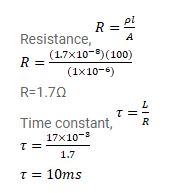# An inductor-coil of inductance 17mH is constructed by

Question:

An inductor-coil of inductance $17 \mathrm{mH}$ is constructed by a copper wire of length $100 \mathrm{~m}$ and cross-sectional area $1 \mathrm{~mm}{ }^{2}$. Calculate the time constant of the circuit if this inductor is joined across an ideal battery. The resistivity of copper= $1.7 \times 10^{8} \Omega-m$.

Solution: